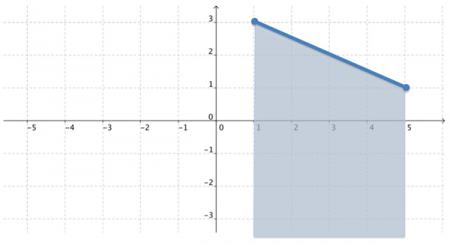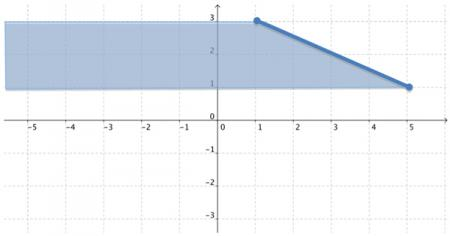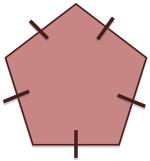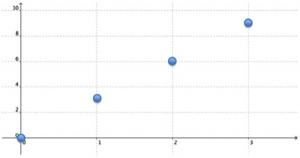# Let's Get Started

We're going learn how to find the domain and range of a graph or verbal description of a situation.

TEKS Standards and Student Expectations

A(2) Linear functions, equations, and inequalities. The student applies the mathematical process standards when using properties of linear functions to write and represent in multiple ways, with and without technology, linear equations, inequalities, and systems of equations. The student is expected to:

A(2)(A) determine the domain and range of a linear function in mathematical problems; determine reasonable domain and range values for real-world situations, both continuous and discrete; and represent domain and range using inequalities

A(6) Quadratic functions and equations. The student applies the mathematical process standards when using properties of quadratic functions to write and represent in multiple ways, with and without technology, quadratic equations. The student is expected to:

A(6)(A) determine the domain and range of quadratic functions and represent the domain and range using inequalities

Resource Objective(s)

Identify mathematical domains and ranges of functions.

Determine reasonable domain and range values for continuous and discrete verbal situations.

Essential Questions

What is the domain of a function and how can it be determined?

What is the range of a function and how can it be determined?

How are continuous functions different from discrete functions?

Vocabulary

# Determine the Domain and Range of a Function

Domain

To determine the domain of a function from a graph, we need to identify the set of all x-coordinates. The x-coordinates on the function's graph tell us about the function's input values.

Let's look at x-values for the graph of a line segment.Notice that the points at either end of the line segment are closed circles. This indicates that these two points are included in the solution.

Also, notice all of the real number points between the closed circles are included, as indicated by the solid line segment. This shows continuous data—data where numbers between any two data values are included in the solution.

In this example, the domain is 1≤ x ≤ 5 since 1 is the smallest x-value and 5 is the biggest x-value.

If the data is not continuous, it is called discrete. An example of discrete data is given later in the resource.

Range

To determine the range of a function from the graph, identify the set of all y-coordinates in the function’s graph. The y-coordinates tell us about the function's output values.

Let's look at the y-values for the same line segment.In this example, the range is 1≤ y ≤ 3 since 1 is the smallest y-value and 3 is the biggest y-value.

Just like with the domain example, the points at either end of the line segment are closed circles.  This indicates that these two points are included in the solution.

# Domain and Range from Verbal Relationships

You can also examine verbal relationships and learn how to determine the domain and/or range given a specific situation.

Example 1

The perimeter of a regular pentagon is 30 centimeters or less. Identify the set to describe ℓ, the length of each side of the pentagon.Since a pentagon has five sides, we know the perimeter will be 5 times ℓ or P = 5ℓ.

Earlier in the resource, we learned the domain is related to the input and the range is related to the output. For this example, the input is the length and the output in the perimeter.

To find the domain, we need to know all the possible values for ℓ that will give us a perimeter less than or equal to 30 centimeters.

We already know that distance is always greater than 0. So ℓ  > 0. We also know that the perimeter is 30 centimeters or less. So 5ℓ ≤ 30.

By solving the inequality 5ℓ ≤ 30, we find the longest length possible is 6 because 5 times 6 is 30. So ℓ ≤ 6.

Our final answer needs to be written in set notation because we were asked to identify the set to describe ℓ. So our answer will be {0

Example 2The number of tennis balls in n cans can be expressed by the function s = 3n. What is the domain and range of this function?

According to the function, the number of cans we have will determine the number of tennis balls. So the input/independent variable is n, and the output/dependent variable is s.

Let’s make a graph to see what happens.

In this example we don’t have things like 1.5 cans of tennis balls. It is 1 can, 2 cans, 3 cans, and so on. Since we can’t use the values between 1 and 2, we say this is a discrete function.

As a graph, this relationship would look like this:What are the domain and range for this function? Click below to check your answer.